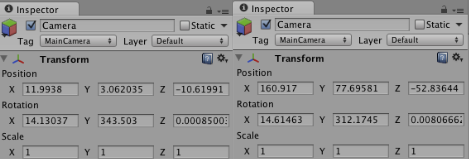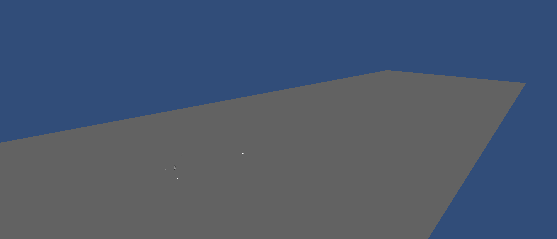# Camera does not follow the object?

Hi,

i am trying to make the camera follow the ball with an offset ( the distance between the ball and the camera at the beginning ). But the camera goes far behind its original position (it kind of follows somehow the movements, but way too far from the ball), i don’t understand why. Here are the coords (on the left “before” : X = 11, Y=3, Z=-10, on the right “after” : X=160, Y = 77, Z = -52)And instead of being close to the ball, the camera is far away from it :Here is the code :

``````//empty.js
if (ballThrown){

if (startPoint != thisTransform.position && !stopToReceiver){
camera_script.ball = thisTransform.position;
camera_script.ballbool = true;
} else {
camera_script.ballbool = false;
}

//ball.js
function Update () {
if (ballbool) {
thisTransform.position = thisTransform.position + ball.position;

thisTransform.rotation = Quaternion.Lerp(thisTransform.rotation, ball.rotation, Time.deltaTime * 5);
}
}
``````

Hi, you can use pre-written script provided by unity package.
Simply put this script on camera and assign target(ball) in inspector.

``````// The target we are following
var target : Transform;
// The distance in the x-z plane to the target
var distance = 10.0;
// the height we want the camera to be above the target
var height = 5.0;
// How much we
var heightDamping = 2.0;
var rotationDamping = 3.0;

function LateUpdate () {
// Early out if we don't have a target
if (!target)
return;

// Calculate the current rotation angles
var wantedRotationAngle = target.eulerAngles.y;
var wantedHeight = target.position.y + height;

var currentRotationAngle = transform.eulerAngles.y;
var currentHeight = transform.position.y;

// Damp the rotation around the y-axis
currentRotationAngle = Mathf.LerpAngle (currentRotationAngle, wantedRotationAngle, rotationDamping * Time.deltaTime);

// Damp the height
currentHeight = Mathf.Lerp (currentHeight, wantedHeight, heightDamping * Time.deltaTime);

// Convert the angle into a rotation
var currentRotation = Quaternion.Euler (0, currentRotationAngle, 0);

// Set the position of the camera on the x-z plane to:
// distance meters behind the target
transform.position = target.position;
transform.position -= currentRotation * Vector3.forward * distance;

// Set the height of the camera
transform.position.y = currentHeight;

// Always look at the target
transform.LookAt (target);
}
``````

Enjoy